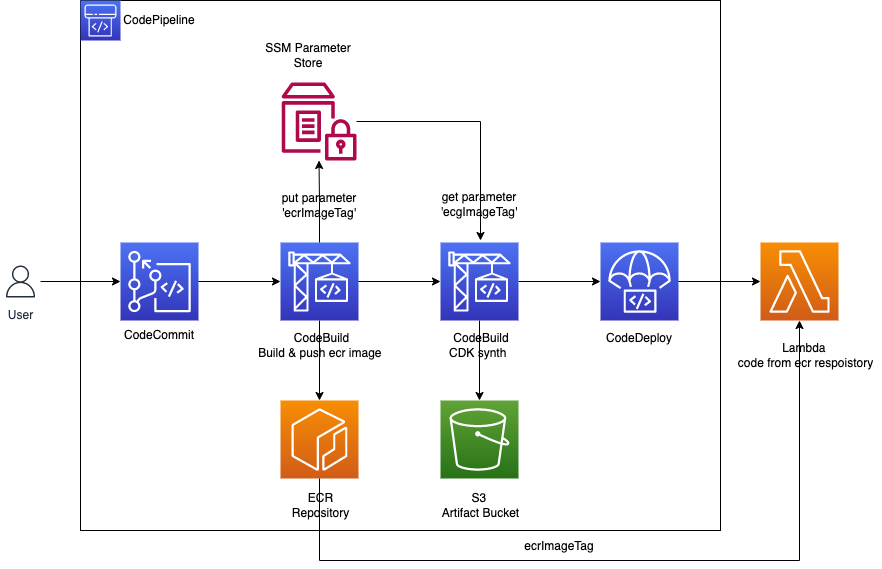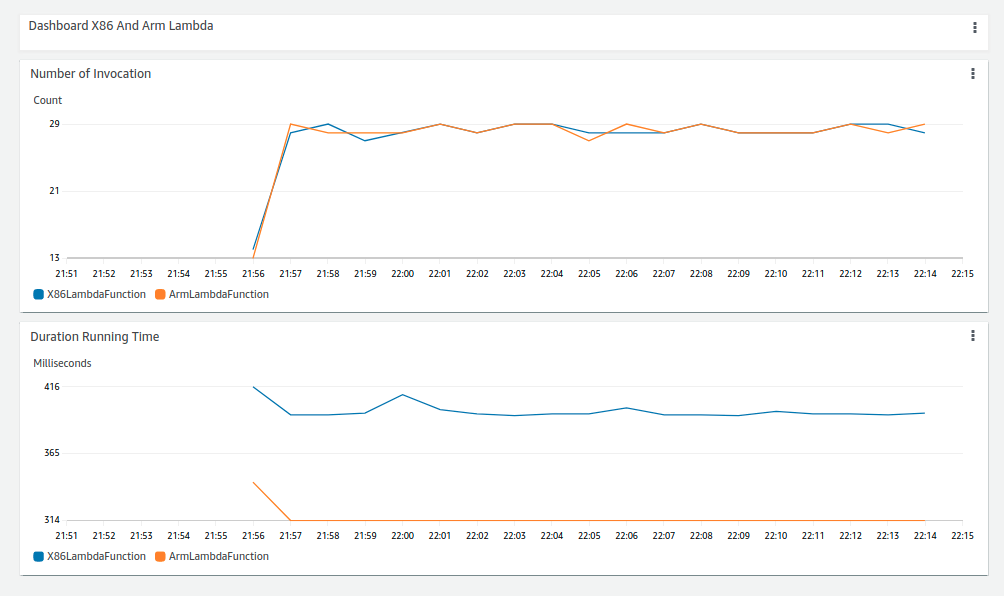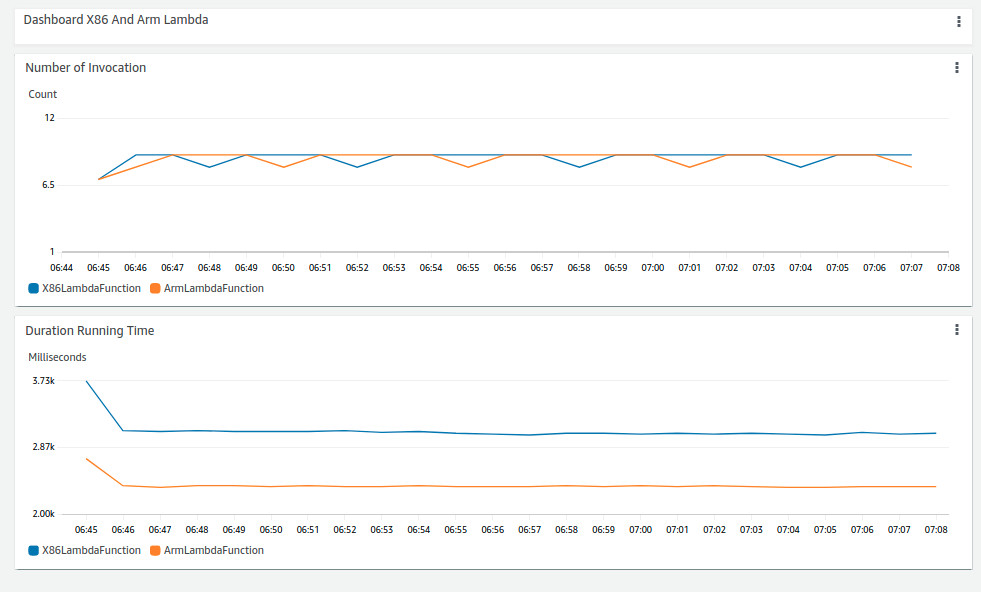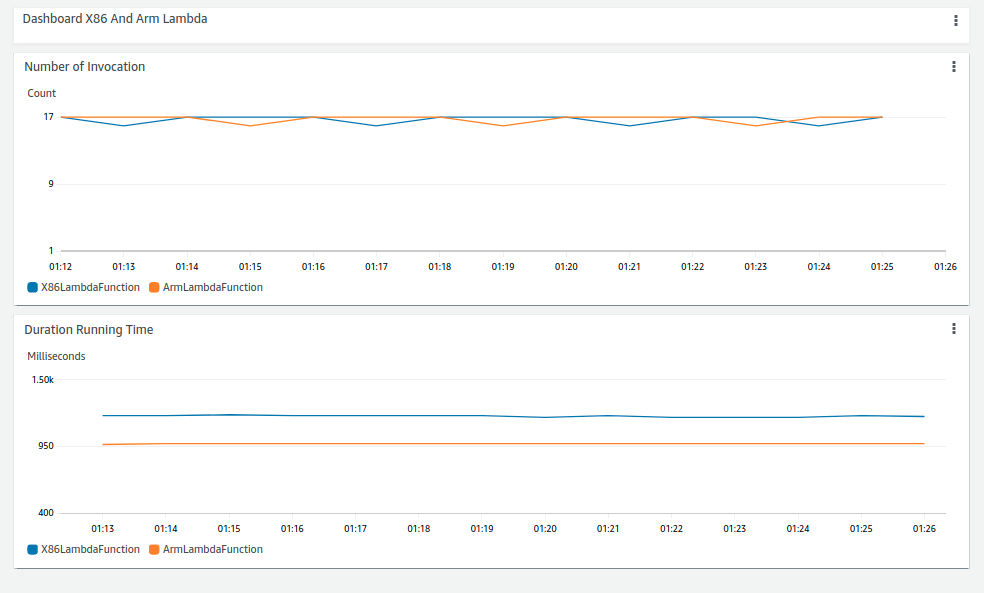According to AWS Graviton2 the Arm Graviton provides 34% better price performance than X86. In some sense, it runs faster 34% compared with X86. So I do a small experiment to check this. AWS CDK and CodeBuild with Arm based instance makes it easy to setup. So the Graviton does run about 30% faster than X86. GitHub

• Runtime Python 3.8
• Numpy 1.22.1
• Lambda memory 2048MB
• Lambda timeout 10 seconds
• Github

## CDK Pipeline#

CDK make it very friendly to build this pipeline, feeling like pure programming infrastructure.## Running time for Fibonaci#

`.css-zbg2gu{-webkit-padding-start:var(--chakra-space-5);padding-inline-start:var(--chakra-space-5);-webkit-padding-end:var(--chakra-space-5);padding-inline-end:var(--chakra-space-5);}def recur_fibo(n):    if n <= 1:        return n    else:        return(recur_fibo(n-1) + recur_fibo(n-2))`

n = 30 used for testing.## Running time for FFT single thread#

`data = np.random.rand(8192, 8192)np.fft.fft(data, (axis = 0))`## Running time for FFT multi-thread (Lambda memory 10240MB)#

`data = [np.random.rand(8192, 2048) for k in range(4)]with ThreadPoolExecutor(max_worker=4) as executor:  for x in data:    executor.submit(np.fft.fft, x, axis=0)`